## ANOVA & ANCOVAANOVA

## Basic Goal of ANOVA

Compare the means of groups that have been sampled randomly, to test whether or not they differ.

The different levels of the categorical variable representing different groups are called treatments.

Each observation is called a replicate and is assumed to be independent from all other replicates.

So how do we compare group means?

Confusingly, it's all about variances (hence ANOVA).

## Intuitive Picture of ANOVA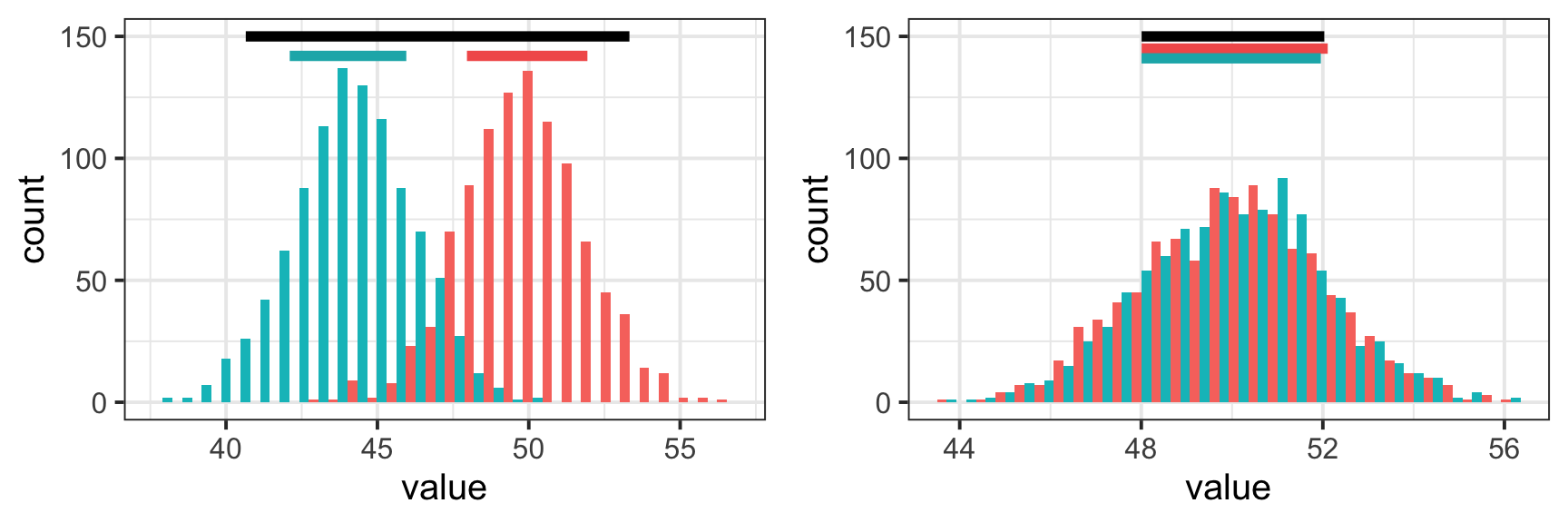Colored bars at top represent variances within groups.

Black bars at top represent variances ignoring groups.

## The ANOVA linear model

$Y_{ij}=\mu + A_i + \epsilon_{ij}$

$$\mu$$ is the population grand mean ($$\bar{Y_{ij}}$$ an unbiased estimator of $$\mu$$)

$$A_i$$ is the additive linear effect compared to the grand mean for a given treatment $$i$$ ($$A_i$$‘s sum to 0).

$$\epsilon_{ij}$$ is the error variance (the variance of individual points around their treatment group means. These are assumed to be distributed as $$N(0,\sigma^2)$$).

## ANOVA linear model - Simulation

$Y_{ij}=\mu + A_i + \epsilon_{ij}$

Let's see how changing model parameters affects the y variable. We will start a few variables that will be kept constant.

grandMean <- 10
groups <- rep(c("A", "B"), 100)
myError <- rnorm(200, mean = 0, sd=3)

## ANOVA linear model - Simulation

y <- grandMean + c(-5, 5) + myError
qplot(groups, y, geom="boxplot", fill=groups)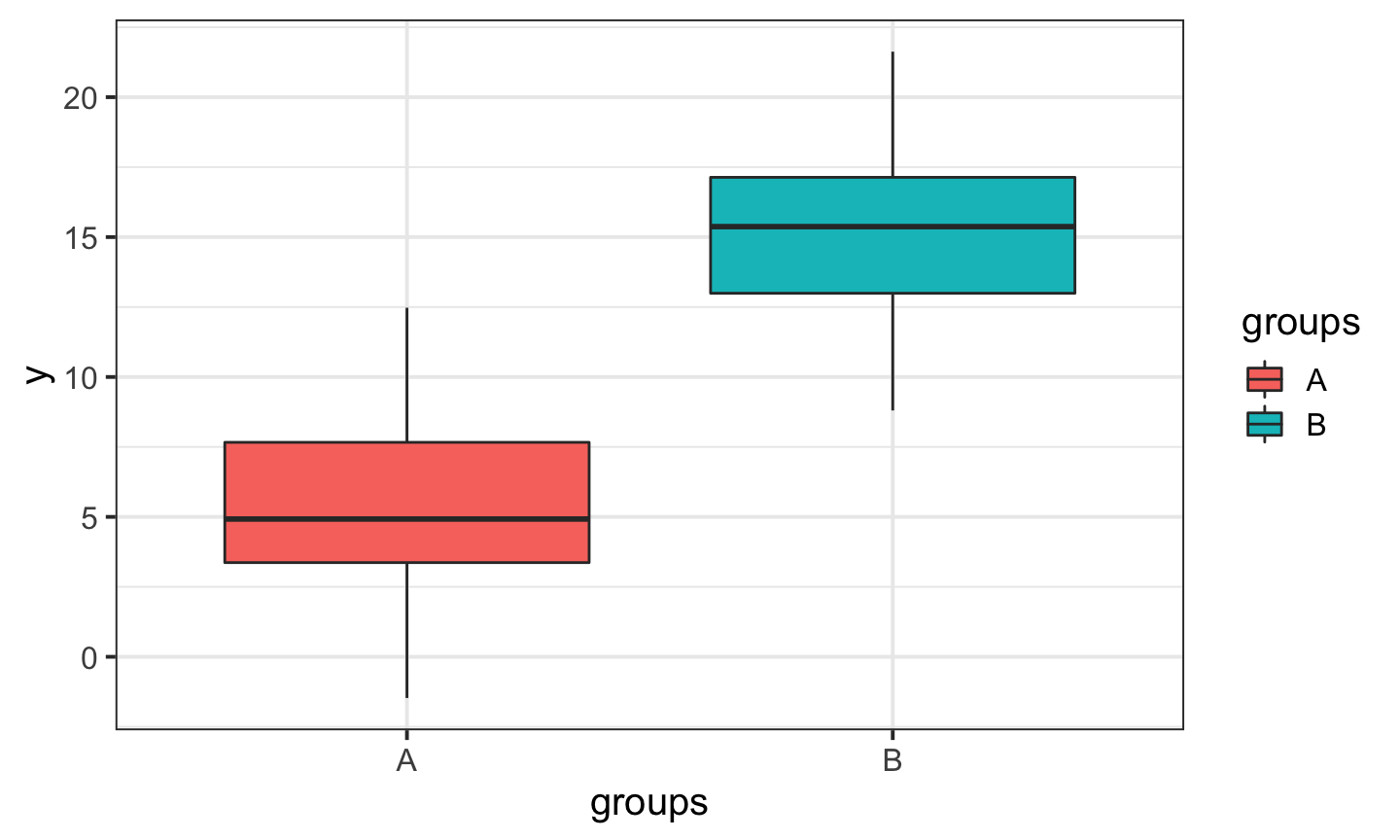## ANOVA linear model - Simulation

y <- grandMean + c(-0.3, 0.3) + myError
qplot(groups, y, geom="boxplot", fill=groups)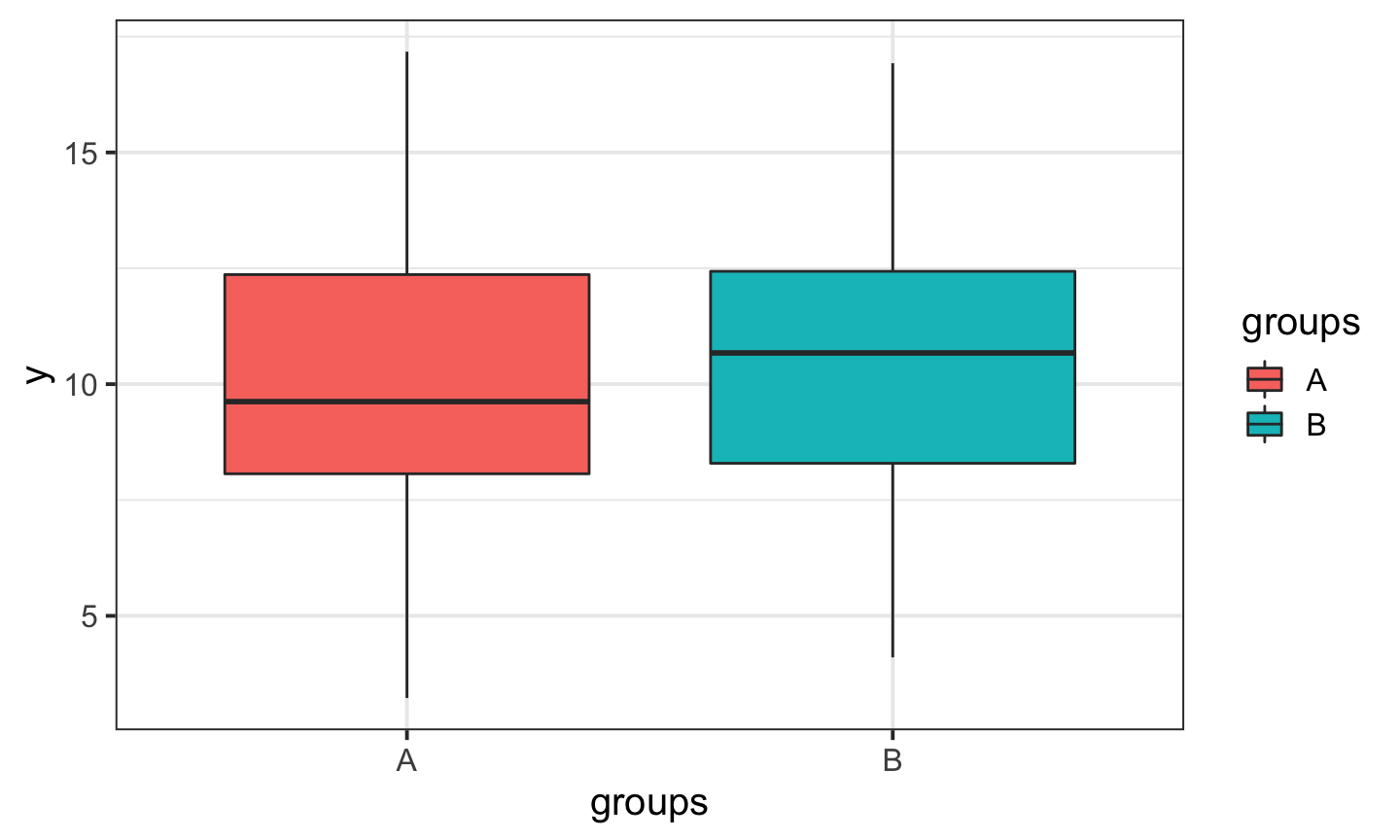## The steps in a one-way ANOVA

1. Calculate the Total SS
2. Calculate the Within-group SS
3. Calculate the Between-group SS
4. Calculate Mean Squares using appropriate df.
5. Calculate the F ratio
6. Calculate the p-value

As you could tell from your reading, calculating the appropriate sum of squares is complicated, and depends on your experimental design. Be careful (or just use Monte Carlo)!

## Walking through the ANOVA

Example data from Gotelli Table 10.1

##   unmanipulated control treatment
## 1            10       9        12
## 2            12      11        13
## 3            12      11        15
## 4            13      12        16

These data represent 12 experimental plots to see the effect of soil temperature on the flowering period in larkspur flowers. The treatment plots had heating coils installed and activated. The control plot had heating coils installed but never turned on. The unmanipulated plots were untouched.

We want to know if there is a difference in mean flowering length between these groups.

## Walking through the ANOVA

Source degrees freedom SS Mean Square (MS) Expected Mean Square F-ratio
Among groups a-1 $$\sum\limits_{i=1}^a\sum\limits_{j=1}^n(\bar{Y}_i - \bar{Y})^2$$ $$\frac{SS_{among_groups}}{(a-1)}$$ $$\sigma^2 + n\sigma^2_A$$ $$\frac{MS_{among}}{MS_{within}}$$
within groups (residual) a(n-1) $$\sum\limits_{i=1}^a\sum\limits_{j=1}^n(Y_{ij} - \bar{Y}_i)^2$$ $$\frac{SS_{within}}{a(n-1)}$$ $$\sigma^2$$
Total an-1 $$\sum\limits_{i=1}^a\sum\limits_{j=1}^n(Y_{ij} - \bar{Y})^2$$ $$\frac{SS_{total}}{(an-1)}$$ $$\sigma^2_Y$$

## Assumptions of ANOVA

1. The samples are independent and identically distributed
2. The residuals $$\epsilon_i$$ are normally distributed
3. Within-group variances are similar across all groups (‘homoscedastic”)
4. Observations are properly categorized in groups

## Supporting assumptions

1. Main effects are additive (no strong interactions).
2. Balanced design (equal number of observations in all groups). If you are going to do a lot of ANOVA, you need to read about the ANOVA controversy in R and other models for calculating SS that are more appropriate for unbalanced designs.

## ANOVA in R

myMod <- lm(iris$Petal.Length~iris$Species)
summary(myMod)
##
## Call:
## lm(formula = iris$Petal.Length ~ iris$Species)
##
## Residuals:
##    Min     1Q Median     3Q    Max
## -1.260 -0.258  0.038  0.240  1.348
##
## Coefficients:
##                        Estimate Std. Error t value Pr(>|t|)
## (Intercept)             1.46200    0.06086   24.02   <2e-16 ***
## iris$Speciesversicolor 2.79800 0.08607 32.51 <2e-16 *** ## iris$Speciesvirginica   4.09000    0.08607   47.52   <2e-16 ***
## ---
## Signif. codes:  0 '***' 0.001 '**' 0.01 '*' 0.05 '.' 0.1 ' ' 1
##
## Residual standard error: 0.4303 on 147 degrees of freedom
## Multiple R-squared:  0.9414, Adjusted R-squared:  0.9406
## F-statistic:  1180 on 2 and 147 DF,  p-value: < 2.2e-16

## ANOVA in R

anova(myMod)
## Analysis of Variance Table
##
## Response: iris$Petal.Length ## Df Sum Sq Mean Sq F value Pr(>F) ## iris$Species   2 437.10 218.551  1180.2 < 2.2e-16 ***
## Residuals    147  27.22   0.185
## ---
## Signif. codes:  0 '***' 0.001 '**' 0.01 '*' 0.05 '.' 0.1 ' ' 1

## Checking assumptions

plot(iris$Petal.Length ~ iris$Species)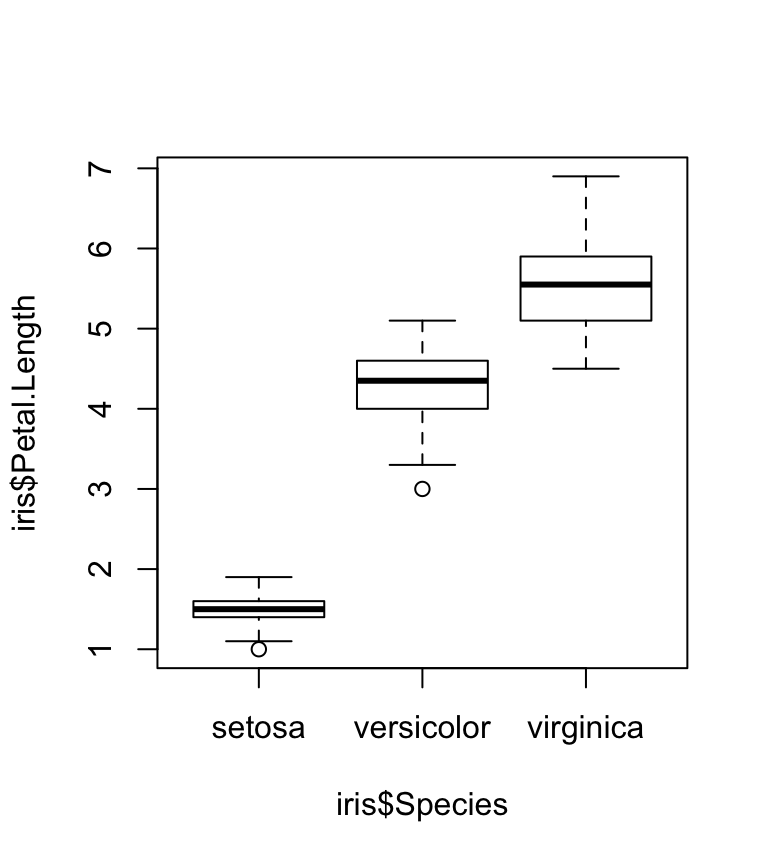#Homogeneity of variances
bartlett.test(iris$Petal.Length ~ iris$Species)
##
##  Bartlett test of homogeneity of variances
##
## data:  iris$Petal.Length by iris$Species
## Bartlett's K-squared = 55.423, df = 2, p-value = 9.229e-13

## Checking assumptions

plot(myMod)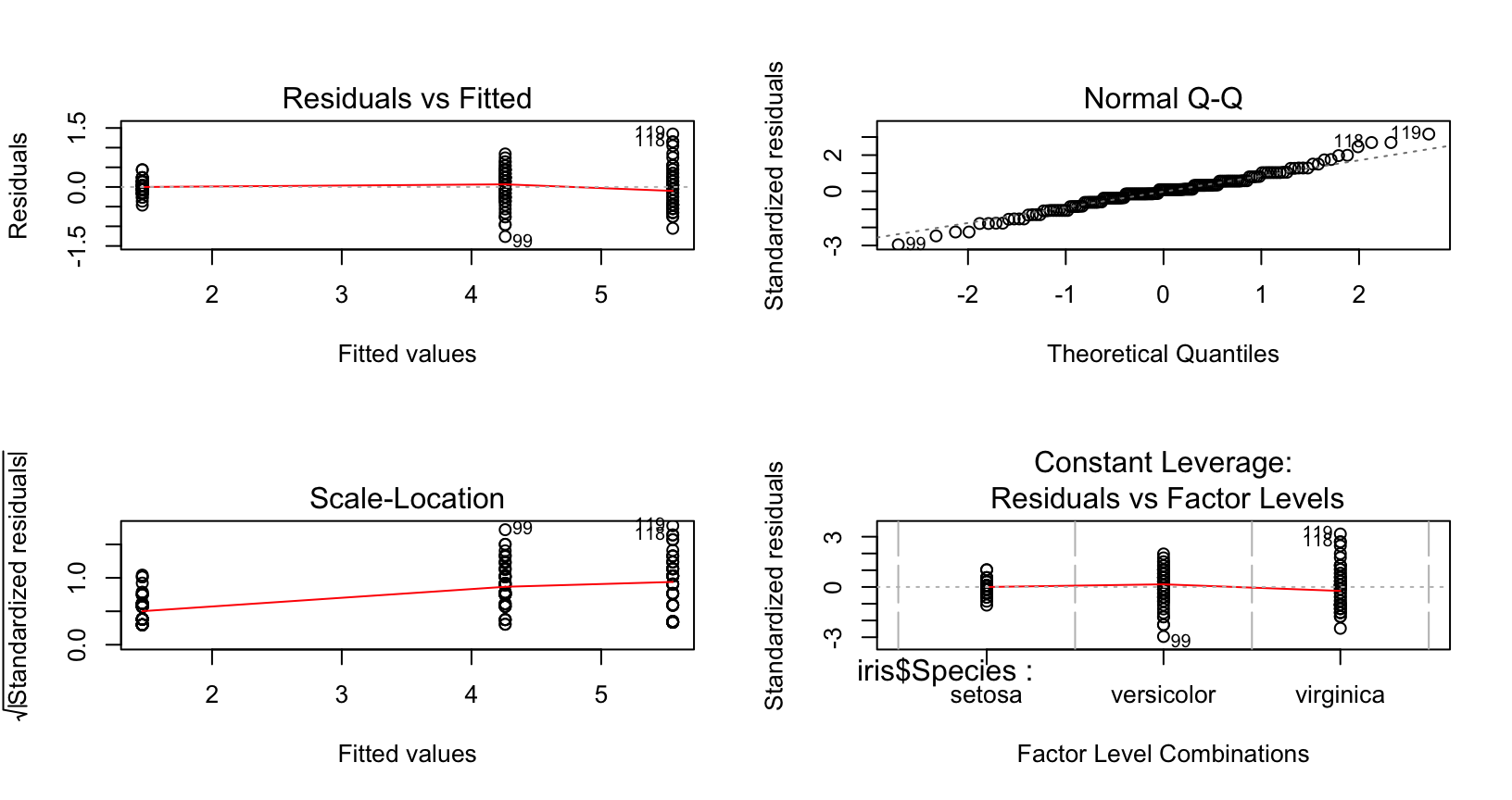## Two-Way ANOVA

Life gets more complicated when we are considering more than one factor

$Y_{ij}=\mu + A_i + B_j + AB_{ij} + \epsilon_{ij}$

With more than one factor, we have the possibility of non-additive effects of one factor on the other. These are called interaction terms

## Simple model (no interaction)

data(warpbreaks)
model <- lm(warpbreaks$breaks ~ warpbreaks$tension + warpbreaks$wool) anova(model) ## Analysis of Variance Table ## ## Response: warpbreaks$breaks
##                    Df Sum Sq Mean Sq F value   Pr(>F)
## warpbreaks$tension 2 2034.3 1017.13 7.5367 0.001378 ** ## warpbreaks$wool     1  450.7  450.67  3.3393 0.073614 .
## Residuals          50 6747.9  134.96
## ---
## Signif. codes:  0 '***' 0.001 '**' 0.01 '*' 0.05 '.' 0.1 ' ' 1

## Two-way ANOVA

Have possibility of non-additive effects, where value of factor A influences value of factor B